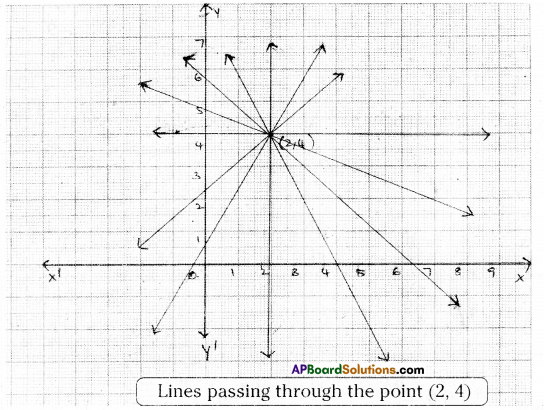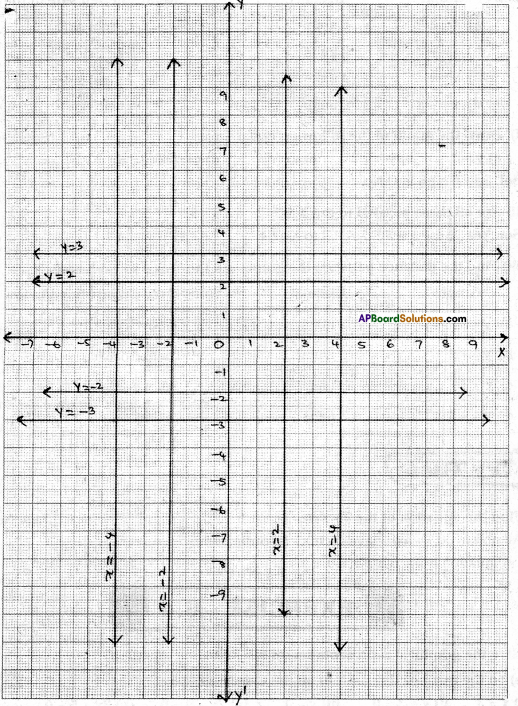AP State Syllabus AP Board 9th Class Maths Solutions Chapter 6 Linear Equation in Two Variables InText Questions and Answers.

## AP State Syllabus 9th Class Maths Solutions 6th Lesson Linear Equation in Two Variables InText Questions

Try This

Question 1.
Express the following linear equations in the form of ax + by + c = 0 and indicate the value of a, b, c in each case. [Page No. 128]
i) 3x + 2y = 9
Solution:
3x + 2y = 9
⇒ 3x + 2y – 9 = 0
Here a = 3, b = 2, c = -9ii) – 2x + 3y = 6
Solution:
– 2x + 3y = 6
⇒ – 2x + 3y – 6 = 0
Here a = -2, b = 3, c = -6

iii) 9x – 5y = 10
Solution:
9x- 5y = 10
⇒ 9x- 5y – 10 = 0
Here a = 9, b = -5, c = -10

iv) $$\frac{x}{2}-\frac{y}{3}-5=0$$
⇒ $$\frac{3 x-2 y-30}{6}=0$$
⇒ 3x – 2y – 30 = 0
Here a = 3, b = – 2 and c = – 30v) 2x = y
Solution:
2x = y
⇒ 2x – y = 0
Here a = 2, b = – 1 and c = 0

Try These

Take a graph paper, plot the point (2, 4), and draw a line passing through it. Now answer the following questions. [Page No. 135]

Question 1.
Can you draw another line that passes through the point (2, 4) ?
Solution:
Yes, We can draw another line passing through (2, 4).

Question 2.
How many such lines can be drawn ?
Solution:
Infinitely many lines can be drawn.Question 3.
How many linear equations in two variables exist for which (2, 4) is a solution ? Solution:
Infinitely many linear equations in two variables exist.
Graph is given in next page.Do This

Question 1.
i) Draw the graph of following equations. [Page No. 145]
a) x = 2 b) x = -2 c) x = 4 d) x = -4ii) Are the graphs of all these equations parallel to Y-axis? .
Solution:
Yes; all these lines are parallel to Y-axis.

iii) Find the distance between the graph and the Y-axis in each case.
Solution:

 Graph DIstance Right side/left side of the origin a) x = 2 2 units Right side b) x = -2 2 units Left side c) x = 4 4 units Right side d) x = -4 4 units Left side

Question 2.
i) Draw the graph of the following equations. [Page No. 145]
a) y = 2 b) y = -2 c) y = 3 d) y = – 3
Solution:ii) Are all these parallel to the X-axis?
Solution:
Yes, these are all parallel to X-axis.

iii) Find the distance between the graph and the X-axis in each case.

 Graph DIstance side of the origin above/below a) y = 2 2 units above origin b) y = -2 2 units below origin c) y = 3 3 units above origin d) y = -3 3 units below originTry These

Question
Find the equation of the Y – axis. [Page No. 146]
Solution:
All points on Y – axis have their x – co-ordinates as zero.
∴ Equation of Y- axis is x = O.